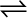7.11 A sample of HI(g) is placed in flask at a pressure of 0.2 atm. At equilibrium the partial pressure of HI(g) is 0.04 atm. What is Kp for the given equilibrium ?

2HI (g)H2 (g) + I2 (g)

The initial concentration of HI is 0.2 atm. At equilibrium, it has a partial pressure of 0.04 atm. Therefore, a decrease in the pressure of HI is 0.2 –

0.04 = 0.16. The given reaction is:

Hence, the value of ${K}_{p}$ for the given equilibrium is 4.0.